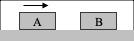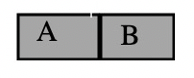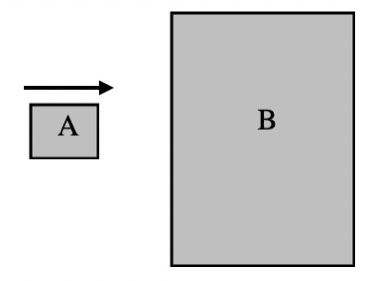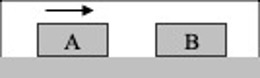Interactive Lecture Demonstration

Prediction Sheet—Momentum

 Demonstration 1: A ball is dropped to the ground.  On the diagram to the right, draw the force(s) acting on the ball as it is falling. Is there a net force acting on the ball as it falls?   Is the momentum of the ball conserved (constant) as it falls?     If you think momentum is conserved, state why.  If not, describe a system, that includes the ball, in which the total momentum is conserved.     Only after you have made your predictions click here to observe the results. Compare to your predictions and explain any differences. (The ball is in the air, falling)Demonstration 2 A ball is dropped to the ground. The ball hits the ground and bounces up. It has the same speed just before and just after it hits the ground.  On the diagram to the right, draw the force(s) acting on the ball while it is in contact with the ground. Is there a net force acting on the ball when it is in contact with the ground?   Is the momentum of the ball conserved (constant) from just before it hits the ground until just after it leaves the ground?   If you think momentum is conserved, state why.  If not, describe a system, that includes the ball, in which the total momentum is conserved.     Only after you have made your predictions click here to observe the results. Compare to your predictions and explain any differences. (The ball is in contact with the ground, bouncing.)Demonstration 3: Two objects A and B, of equal mass, move towards each other. Assume that friction is so small that it may be neglected. The objects have the same initial speed. They collide and bounce apart. In the lower half of the box on the right, draw the force(s) acting on each object as they collide (while they are in contact with each other). Compare their magnitudes.   Compare the momentum of either object just before the collision to its momentum just after the collision.   Compare the total (combined) momentum of the two objects before the collision to their total momentum after the collision.   Only after you have made your predictions, click here to view the forces. Then open the Collision Lab simulation: https://phet.colorado.edu/sims/collision-lab/collision-lab_en.html Click on More Data, and make the masses equal and make velocity2 the negative of velocity1. Click the checkbox Show Values and unclick Velocity Vectors. Be sure that the slide bar is set all the way to the right for an Elastic collision. Click Play to run the simulation. You can click Pause to stop or Restart and Play to run the simulation again.   Compare your results to your predictions. Explain any differences.     Was momentum conserved? Explain.Demonstration 4: Two objects A and B, of equal mass, collide and bounce apart. One of the objects is initially traveling to the right, and the other object is initially at rest. In the lower half of the box on the right, draw the force(s) acting on each object as they collide (while they are in contact with each other). How do the magnitudes of these forces compare? Compare the momentum of either object just before the collision to its momentum just after the collision.   Compare the total (combined) momentum of the two objects before the collision to their total momentum after the collision.   Only after you have made your predictions, click here to view the forces. Then use the same Collision Lab simulation. First click Restart. Be sure the masses are equal and make velocity2 zero. The slide bar should again be set all the way to the right: Elastic collision. Click Play to run the simulation. You can click Pause to stop or Restart and Play to run the simulation again.   Compare your results to your predictions. Explain any differences.     Was momentum conserved? Explain.Demonstration 5: Two objects A and B, of equal mass, collide and stick together. One of the objects is initially traveling to the right, and the other object is initially at rest. In the lower half of the box on the right, draw the force(s) acting on each object as they collide (while they are in contact with each other). How do the magnitudes of these forces compare? Compare the momentum of either object just before the collision to its momentum just after the collision.   Compare the total (combined) momentum of the two objects before the collision to their total momentum after the collision.   Only after you have made your predictions, click here to view the forces. Then use the same Collision Lab simulation. First click Restart. Be sure the masses are equal and make velocity2 zero. The slide bar should now be set all the way to the left: Inelastic collision. Click Play to run the simulation. You can click Pause to stop or Restart and Play to run the simulation again.   Compare your results to your predictions. Explain any differences.     Was momentum conserved? Explain.Demonstration 6: A small object collides with a much more massive object initially at rest and bounces off of it. Assume that friction is so small that it may be neglected. In the box to the right, draw the force(s) acting on each object as they collide (while they are in contact with each other). How do the magnitudes of these forces compare?   Is the momentum of the small object conserved?   Is the momentum of the large object conserved?   Is the combined momentum of the two objects conserved?   Only after you have made your predictions, click here to view the forces. Then use the same Collision Lab simulation. First click Restart. Make    Mass 1 0.05 kg and Mass 2 50 kg. Make velocity1 1 m/s and make velocity2 zero. The slide bar should now be set all the way to the right: Elastic collision. Click Play to run the simulation. You can click Pause to stop or Restart and Play to run the simulation again.   Compare your results to your predictions. Explain any differences.     Was momentum conserved? Explain.